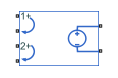# PCCVS2

Polynomial current-controlled voltage source with two controlling inputs

•Libraries:
Simscape / Electrical / Additional Components / SPICE Sources

## Description

The PCCVS2 (Two-Input Polynomial Current-Controlled Voltage Source) block represents a voltage source whose output voltage value is a polynomial function of the currents through the pairs of controlling input ports. This equation describes the voltage across the source as a function of time:

`${V}_{out}={p}_{1}+{p}_{2}*{I}_{in1}+{p}_{3}*{I}_{in2}+{p}_{4}*{I}_{in1}^{2}+{p}_{5}{I}_{in1}*{I}_{in2}+{p}_{6}*{I}_{in2}^{2}+\dots$`

where:

• Iin1 is the current through the first pair of input ports.

• Iin2 is the current through the second pair of input ports.

• p is the Polynomial coefficients parameter value.

## Ports

### Conserving

expand all

Electrical conserving port associated with the PCCVS2 positive voltage of the first controlling input.

Electrical conserving port associated with the PCCVS2 negative voltage of the first controlling input.

Electrical conserving port associated with the PCCVS2 positive voltage of the second controlling input.

Electrical conserving port associated with the PCCVS2 negative voltage of the second controlling input.

Electrical conserving port associated with the PCCVS2 positive output voltage.

Electrical conserving port associated with the PCCVS2 negative output voltage.

## Parameters

expand all

The polynomial coefficients that relate the input current to the output voltage, as described in the preceding section.

## Version History

Introduced in R2009a